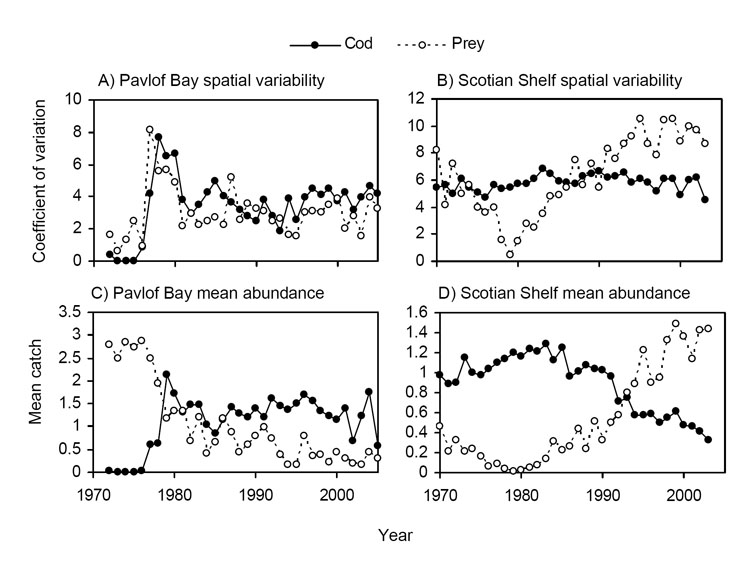### Michael A. Litzow, J. Daniel Urban, and Benjamin J. Laurel. 2008. Increased spatial variance accompanies reorganization of two continental shelf ecosystems. Ecological Applications 18:1331–1337.

Appendix A. A table presenting the detection ability of shifts in ecosystem variance (Table A1) and a figure showing the spatial variance and mean abundance of cod and prey in the Scotian Shelf and Pavlof Bay time series (Fig. A1).

TABLE A1. Sensitivity of detection of shifts in ecosystem variance and mean state to analytical assumptions. Results from sequential t tests for analysis of regime shifts (STARS) are determined by the designated cut-off length (L) of proposed regimes and the Huber weight parameter (H), the critical distance (in standard deviations) from regime means at which observations are treated as outliers. The year of the first shift in variance and mean values is identified for all combinations of L = 5 and 10 years and H = 1, 2, and 3 in both ecosystems. STARS consistently detected shifts in variance before shifts in mean state, with the exception that the increase in variance associated with the Pavlof Bay phase transition was too short to be detected at L = 10 years.

 Variance shift Mean shift Ecosystem L (years) H (SD) Year P Year P Pavlof Bay 5 1 1977 0.003 1978 < 0.0001 5 2 1977 0.003 1978 < 0.0001 5 3 1977 0.003 1978 < 0.0001 10 1 - - 1979 < 0.0001 10 2 - - 1979 < 0.0001 10 3 - - 1979 < 0.0001 Scotian Shelf 5 1 1989 < 0.0001 1992 < 0.0001 5 2 1989 < 0.0001 1992 < 0.0001 5 3 1989 < 0.0001 1992 < 0.0001 10 1 1989 < 0.0001 1992 < 0.0001 10 2 1989 < 0.0001 1992 < 0.0001 10 3 1989 < 0.0001 1992 < 0.0001FIG. A1. Individual time series of spatial variance and mean abundance for cod and prey as measured by trawl surveys of Pavlof Bay (1972–2005) and the Scotian Shelf (1970–2003). (A) spatial variability in Pavlof Bay, (B) spatial variability on the Scotian Shelf, (C) mean abundance in Pavlof Bay, and (D) mean abundance on the Scotian Shelf. Units on the y-axis are kg / km towed in (C) and individuals / set in (D).

[Back to A018-047]